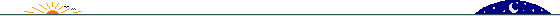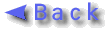Predrag Stojkov Publications• "New Approximations to the Fradkin representation for Green's functions"
• Predrag L. Stojkov
• hep-th/9706135 ( http://xxx.lanl.gov/abs/hep-th/9706135 )
• Submitted to Phys. Rev. D
• RevTeX , 22 pages

A new variant of the exact Fradkin representation of the Green's function $G_c(x,y|gU)$, defined for arbitrary external potential $U$, is presented. Although this new approach is very similar in spirit to that previously derived by Fried and Gabellini, for certain calculations this specific variant, with its prescribed approximations, is more readily utilizable.

Application of the simplest of these forms is made to the $\lambda\Phi^4$ theory in four dimensions. As an independent check of these approximate forms, an improved version of the Schwinger-DeWitt asymptotic expansion of parametrix function is derived.

• "Symmetries of the two-wave mixing in photoreactives"
• Predrag Stojkov, Dejan Timotijevi\'c and Milivoj Beli\'c
• Optic Letters, Vol. 17, N20, pp. 1406-1408; 1992

Symmetries of two-wave mixing equations in photorefractive crystals are considered using group theoretical methods. Symmetry groups for both the equations and conserved quantities are determined, and the corresponding generators are written down explicitly. result obtained for the plane-parallel polarized two-wave mixing are used to introduce the method for solution and the form of solutions for the cross polarized two-wave mixing

• "Symmetries of the photoreactive four-wave mixing"
• Predrag Stojkov and Milivoj Beli\'c
• Phys. Rev. A, Vol. 45, N7, 1 April 1992, P.5061-5064
• Republished in Landmark Papers on Photorefractive nonlinear Optics,
Editors: Pochi Yeh and Claire Gu; World Scientific; 1995; pp 281-284;
ISBN 981021443 X ; Qc446.2.L36 1995

A symmetry analysis of degenerate four-wave mixing equations in photorefractive crystals is carried out. Using underlying Su-symmetries, a systematic derivation of conserved quantities is performed, and a new method of integration of the equations introduced. Five conserved quantities are found, suggesting that the initial four complex equations can be expressed in terms of three real quantities (Euler angles or other). However,. due to the form of equations, only one independent variable is found necessary to solve the problem.

• "Chaos in optical phase conjugation"
• M.R. Beli\'c, W.Krolikowski and P.Stojkov
• Nonlinear World , p. 387-398 , World Scientific ; IV International Workshop on Nonlinear and Turbulent Processes in Physics 9-22 October 1989. Kiev, USSR

We have investigated instabilities in optical phase conjugation arising in different geometrical arrangements, and driven by different control parameters. First a single photorefractive crystal has been considered, with the conjugation achieved through a single region four wave mixing. It is shown (by numerical integration of slowly varying envelope equations) that the phase conjugate output becomes chaotic when the system operates above the self-oscillation threshold, and is driven by the input signal. Second, a resonator configuration has been considered, with a thin Kerr-type phase conjugator operating in the Rama-Nath approximation. In this case wave and medium propagation equations are reduced to two dimensional iterative multimodal map, which is driven to chaos by increasing the pump beam intensity. In both cases predominantly Feigenbaum and intermittency chaotic scenarios have been observed.

• "Chaos in phase-conjugate resonators as a multimodal mapping"
• M.R.Beli\'c, P.Stojkov
• Optical and Quantum Electronics 22 (1990) 157-165

An optical phase-conjugate resonator, realised by a Kerr-like phase conjugator, and represented by a two-dimensional slowly varying envelope plane-wave model is driven to chaos using two laser pumps in standard four-wave mixing geometry. The relevant slowly varying envelope wave equations are reduced to a one-dimensional map for intracavity intensity arising in this analysis are considered using elementary symbolic dynamics methods.Updated on June 24th 1997